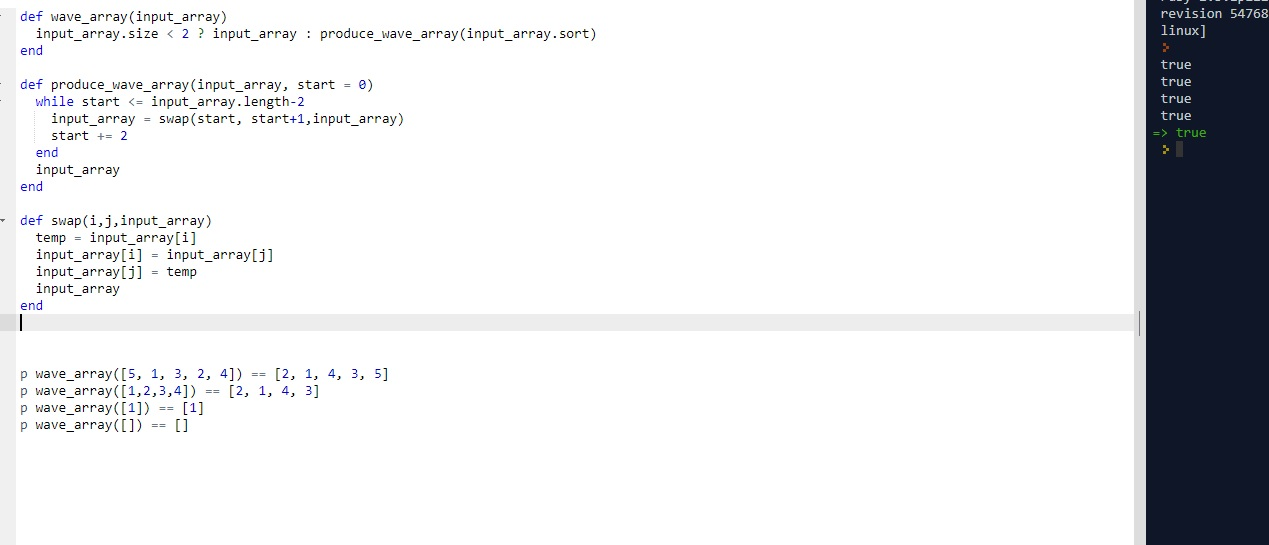# CLEAN CODE: WAVE ARRAY

By: Saurav

2017-12-19 03:52:00 UTC

Given an array of integers, sort the array into a wave like array and return it,
In other words, arrange the elements into a sequence such that a1 >= a2 <= a3 >= a4 <= a5.....

Example

Given [1, 2, 3, 4]

One possible answer : [2, 1, 4, 3]
Another possible answer : [4, 1, 3, 2]
NOTE: If there are multiple answers possible, return the one that's lexicographically smallest.

Interview Bit

Approach:

This is one of those questions where you need to see the pattern as a hint.
The pattern here is:
a1 >= a2 <= a3 >= a4 <= a5.....

Its given we have to sort the array. A normal sort generally sorts an array in a pattern like:
a1<= a2 <= a3 <= a4 <= a5.....

If you see it closely, we see the required pattern can be generated by taking pairs of consecutive array elements in a sorted array and swapping them.

(a1<= a2) <= (a3 <= a4) <= a5.....

After swapping:

(a1 => a2) <= (a3 => a4) <= a5.....

As we see, this approach clearly works. So, let's write few tests first.

```p wave_array([5, 1, 3, 2, 4]) == [2, 1, 4, 3, 5]
p wave_array([1,2,3,4]) == [2, 1, 4, 3]
p wave_array() == 
p wave_array([]) == []```

Lets write our first code:

```def wave_array(input_array)
return input_array if input_array.size < 2

input_array = input_array.sort

start = 0

while start <= input_array.length-2
input_array = swap(start, start+1,input_array)
start += 2
end

return input_array

end

def swap(i,j,input_array)
temp = input_array[i]
input_array[i] = input_array[j]
input_array[j] = temp

return input_array
end
```

Not the most elegant one is it? But looks pretty neat.

Lets do some cleaning:

```def wave_array(input_array)
input_array.size < 2 ? input_array : produce_wave_array(input_array.sort)
end

def produce_wave_array(input_array, start = 0)
while start <= input_array.length-2
input_array = swap(start, start+1,input_array)
start += 2
end
input_array
end

def swap(i,j,input_array)
temp = input_array[i]
input_array[i] = input_array[j]
input_array[j] = temp
input_array
end

```As we see all our tests pass and the code looks pretty neat and each function handles only one level of abstraction as well.

### Let me know what you think! twitter: sprakash24octlinkedin

Owned & Maintained by Saurav Prakash

If you like what you see, you can help me cover server costs or buy me a cup of coffee though donation :)